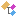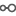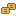﻿ Nevron.FormulaSheet Namespace
Nevron .NET Vision
Inheritance Hierarchy
In This Topic
Nevron.FormulaSheet Namespace
In This Topic
Classes
ClassDescriptionThe NAddCellVerb verb is used for the visual insertion of a named cell in a formula sectionThe NAddRowVerb verb is used for the addition of a new formula section row in a formula sectionThe NBooleanFormulaCell is a formula cell, which has a strong type of BooleanThe NDataGridColumnInfo class encapsulates data grid column information, which a formula section needs to create a data grid for its cells or rows.The NFormulaCell class represents a cell from a formula row or formula section. The formula cell value can be a constant value, or the result of a formula evaluation. The NFormulaCell class serves as base class for strongly typed cells and variant type cell.The NFormulaCellDictionary class represents a dictionary for strongly named formula cellsThe NFormulaRow class represents a collection of cells inside the formula section rows collection.The NFormulaRowCollection class is a strongly typed collection, containing NFormulaRow instancesThe NFormulaSection class represents a category of named formula cells, which can optionally contain a collection of formula rows.The NFormulaSectionCollection class is a strongly typed collection, containing NFormulaSection instancesThe NFormulaSheet class is a container of formula sections, which is used for the formula definition of an object. The NFormulaSheet class serves as base class for more specialized formula sheets.The NFormulaSheetEvaluator class is used for the evaluation of the formula cells inside a formula sheet.The NInsertRowVerb verb is used for the insertion of a new formula section row, after a specific rowThe NInsertRowVerb verb is used for the insertion of a new formula section row, at the place of a specific rowThe NInt32FormulaCell is a formula cell, which has a strong type of Int32Represents a host for a formula cell, which adds a name to the cellThe NRemoveCellsVerb verb is used for the visual removal of named cells from a formula sectionThe NRemoveRowVerb verb is used for the removal of a formula section rowThe NSingleFormulaCell is a formula cell, which has a strong type of SingleThe NStringFormulaCell is a formula cell, which has a strong type of StringThe NStrongTypeOption represents a named value which a strongly typed cell can provide for its enhanced visual editingThe NVariantFormulaCell is a formula cell, which is not strongly typed and stores a variant as its valueThe NVerb class represents a verb of a formula sheet or formula section. It serves as base class for all verbs, which appear in the formula sheet editor.The NVerbCollection class represents a collection of verbs
Interfaces
InterfaceDescriptionImplemented by objects, which host formula sheets
Enumerations
EnumerationDescriptionDefines the possible ways in which a formula sheet can be viewed